# Crates 2

One crate will hold 50 oranges. If Bob needs to ship 932 oranges, how many crates will he need?

Correct result:

n =  19

#### Solution: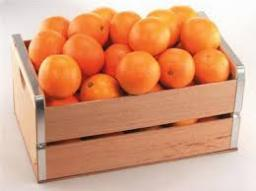We would be pleased if you find an error in the word problem, spelling mistakes, or inaccuracies and send it to us. Thank you!Tips to related online calculators
Do you want to round the number?

## Next similar math problems:

• In fractionsAn ant climbs 2/5 of the pole on the first hour and climbs 1/4 of the pole on the next hour. What part of the pole does the ant climb in two hours?
• Valid numberRound the 453874528 on 2 significant numbers.
• Date game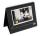Let now is Wednesday. What day is after 248 days? Write the result as a number: 1=Monday, 2=Tuesday, 3=Wednesday, 4=Thursday, 5=Friday, 6=Saturday, 7=Sunday?
• Round 9Round number 0.2375 TO 2 SIGNIFICANT FIGURES
• Round it0.728 round to units, tenths, hundredths.
• A baker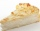A baker has 5 1/4 pies in her shop. She cut the pies in pieces that are each 1/8 of a whole pie. How many pieces of pie does she have?
• Sweets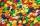Mom bought box of sweets for their children.Whole package of 100 sweets divided among 4 their children so that each child receives the most and for she remains the least sweets. How many sweets left for mom.
• Product of two fractionsProduct of two fractions is 9 3/5 . If one of the fraction is 9 3/7. Find the other fraction.
• Fractions 4How many 2/3s are in 6?
• The resultHow many times I decrease the number 1632 to get the result 24?
• Math classificationIn 3A class are 27 students. One-third got a B in math and the rest got A. How many students received a B in math?
• RemainderWhat is the remainder of the division of natural numbers 293 and 7?
• Pizza 5You have 2/4 of a pizza and you want to share it equally between 2 people how much pizza does each person get?
• RoundingThe following numbers round to the thousandth:
• Yogurt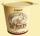The board is 35 yogurts. How many boards of yogurts must be ordered for 886 people in the canteen?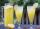How many 1/4 cup servings are in 2 and 1/3 cups of lemonade?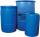When is in 14 barrels 140 liters of water, how many liters barrels of 8 liters I need to get all the water from the larger barrels?